# 数据库面试必问-二叉树和红黑树有什么区别？

2022年12月23日 339

## 红黑树和二叉树有什么区别？

### 二叉树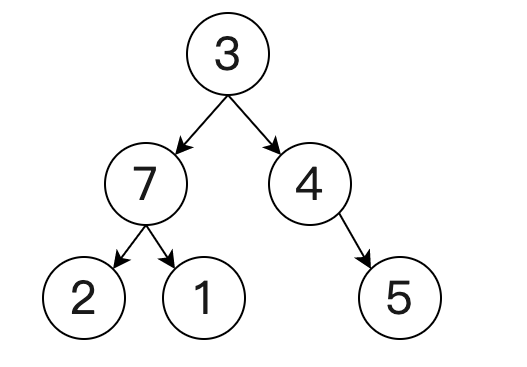• 若任意节点的左子树不为空，则左子树上所有节点的值均小于它的根节点的值；
• 若任意节点的右子树不为空，则右子树上所有节点的值均大于或等于它的根节点的值；
• 任意节点的左、右子树分别为二叉查找树。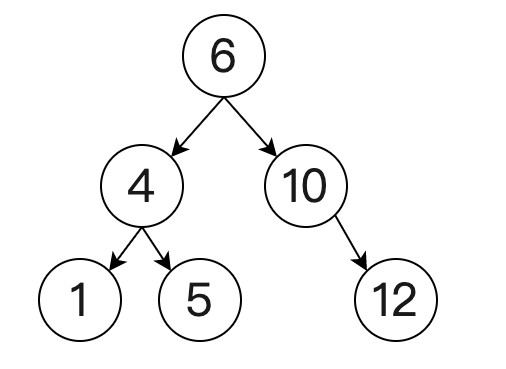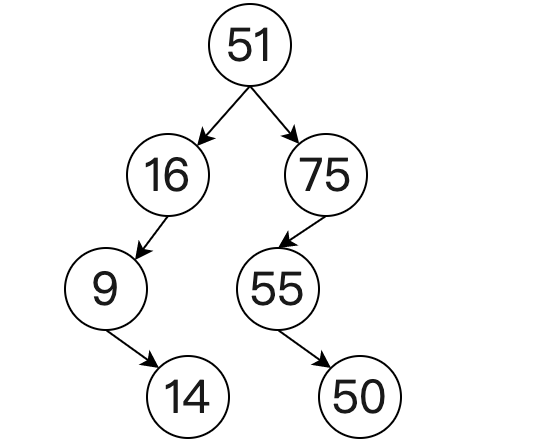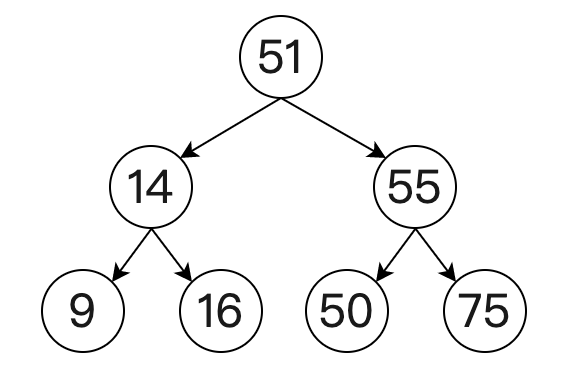### 红黑树

• 节点是红色或黑色；
• 根节点是黑色；
• 所有叶子都是黑色的空节点（NIL 节点）；
• 每个红色节点必须有两个黑色的子节点，也就是说从每个叶子到根的所有路径上，不能有两个连续的红色节点；
• 从一个节点到该节点的子孙节点的所有路径上包含相同数目的黑色节点。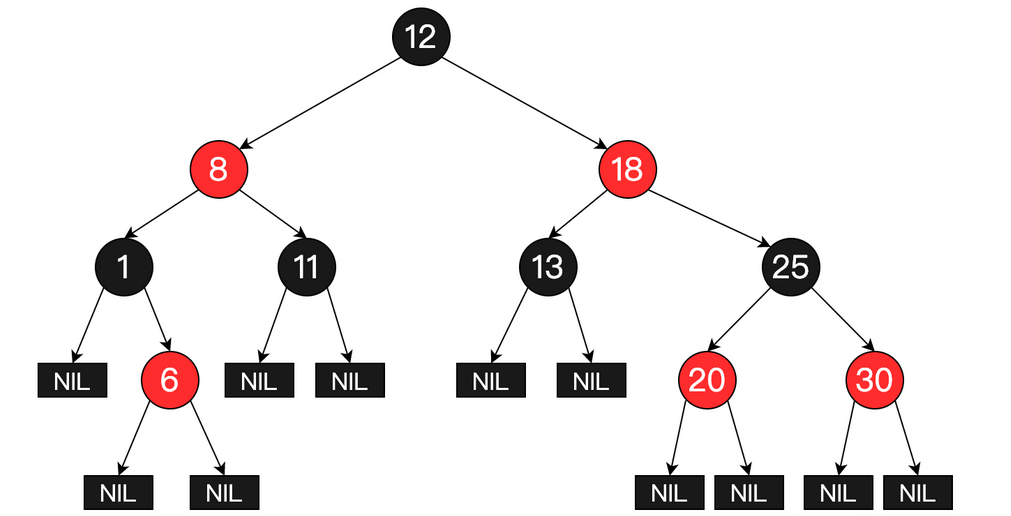## 考点分析

1. 为什么工程中喜欢使用红黑树而不是其他二叉查找树？
2. 红黑树是如何保证自平衡的？

## 知识扩展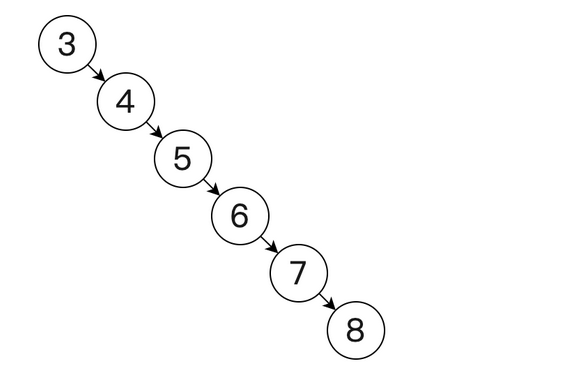• O(1)：常量时间，计算时间与数据量大小没关系；
• O(n)：计算时间与数据量成线性正比关系；
• O(logn)：计算时间与数据量成对数关系；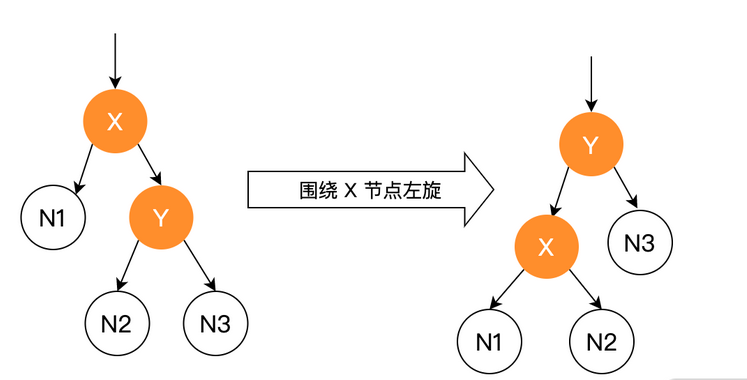```// 源码基于 JDK 1.8 private void rotateLeft(Entry<K,V> p) { if (p != null) { // 右子节点 Entry<K,V> r = p.right; // p 节点的右子节点为 r 的左子节点 p.right = r.left; // r 左子节点如果非空，r 左子节点的父节点设置为 p 节点 if (r.left != null) r.left.parent = p; r.parent = p.parent; // r 父节点等于 p 父节点 // p 父节点如果为空，那么讲根节点设置为 r 节点 if (p.parent == null) root = r; // p 父节点的左子节点如果等于 p 节点，那么 p 父节点的左子节点设置 r 节点 else if (p.parent.left == p) p.parent.left = r; else p.parent.right = r; r.left = p; p.parent = r; } } ```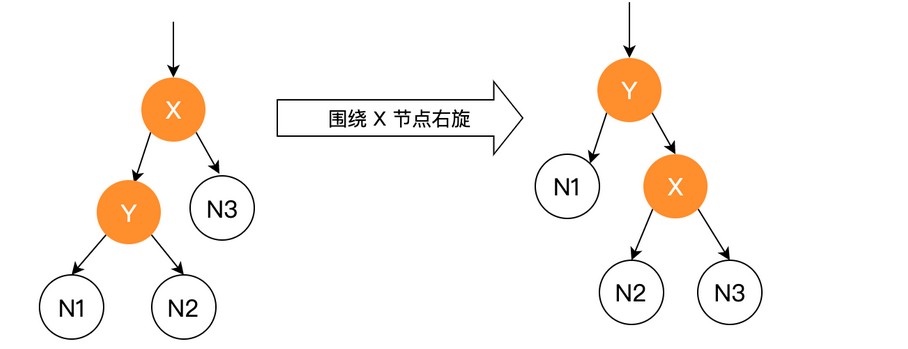```private void rotateRight(Entry<K,V> p) { if (p != null) { Entry<K,V> l = p.left; // p 节点的左子节点为 l 的右子节点 p.left = l.right; // l 节点的右子节点非空时，设置 l 的右子节点的父节点为 p if (l.right != null) l.right.parent = p; l.parent = p.parent; // p 节点的父节点为空时，根节点设置成 l 节点 if (p.parent == null) root = l; // p 节点的父节点的右子节点等于 p 节点时，p 的父节点的右子节点设置为 l else if (p.parent.right == p) p.parent.right = l; else p.parent.left = l; l.right = p; p.parent = l; } } ```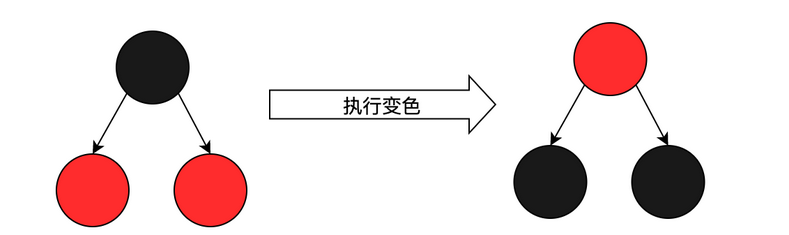## 总结PHP学习网：站内收集的部分资源来源于网络，若侵犯了您的合法权益，请联系我们删除！

### 文章评论

PHP学习网